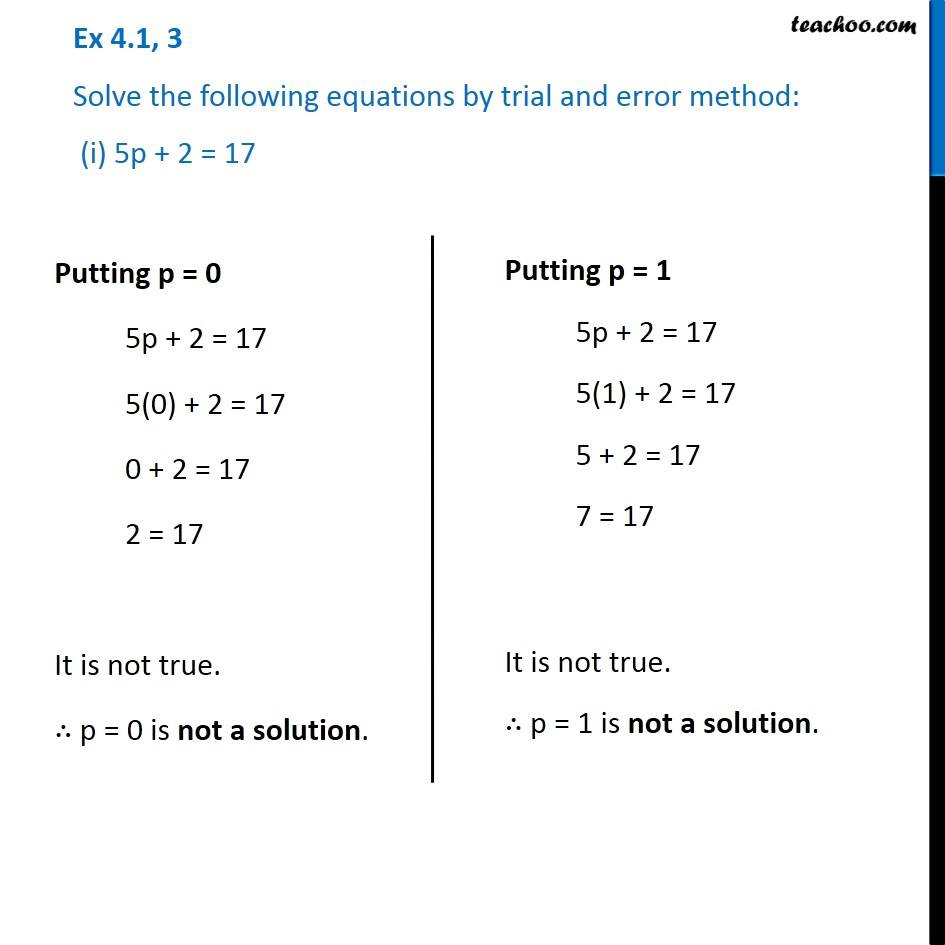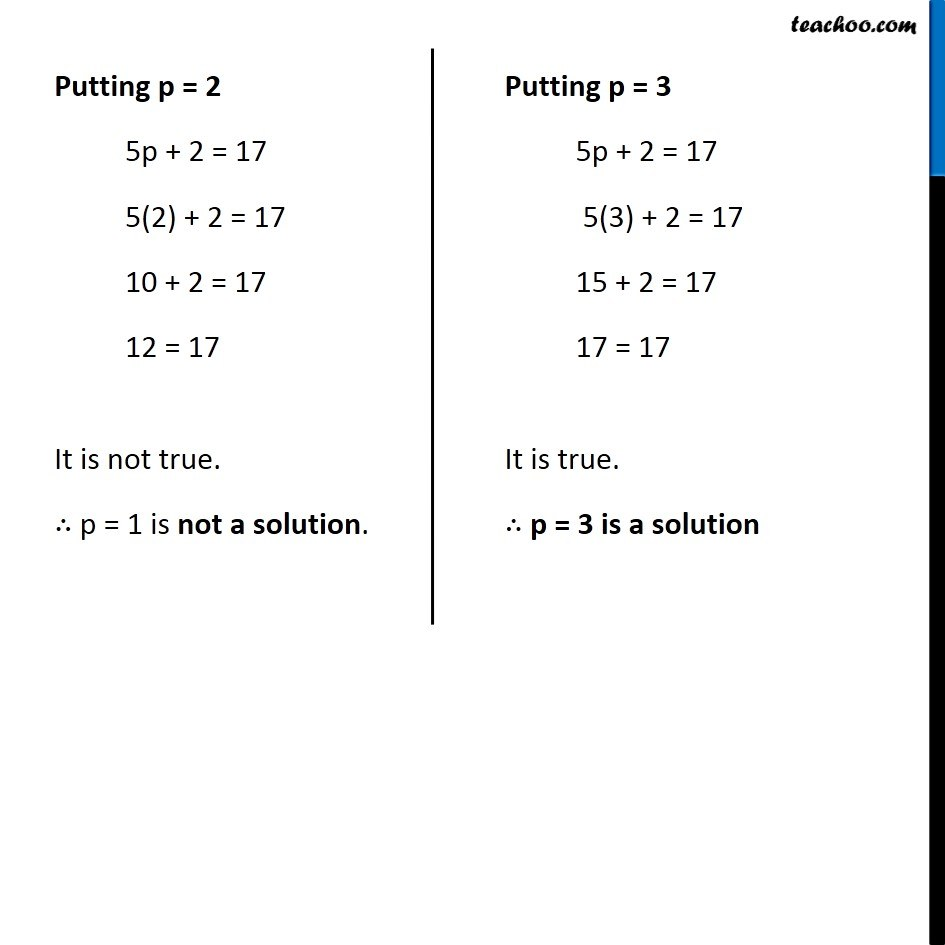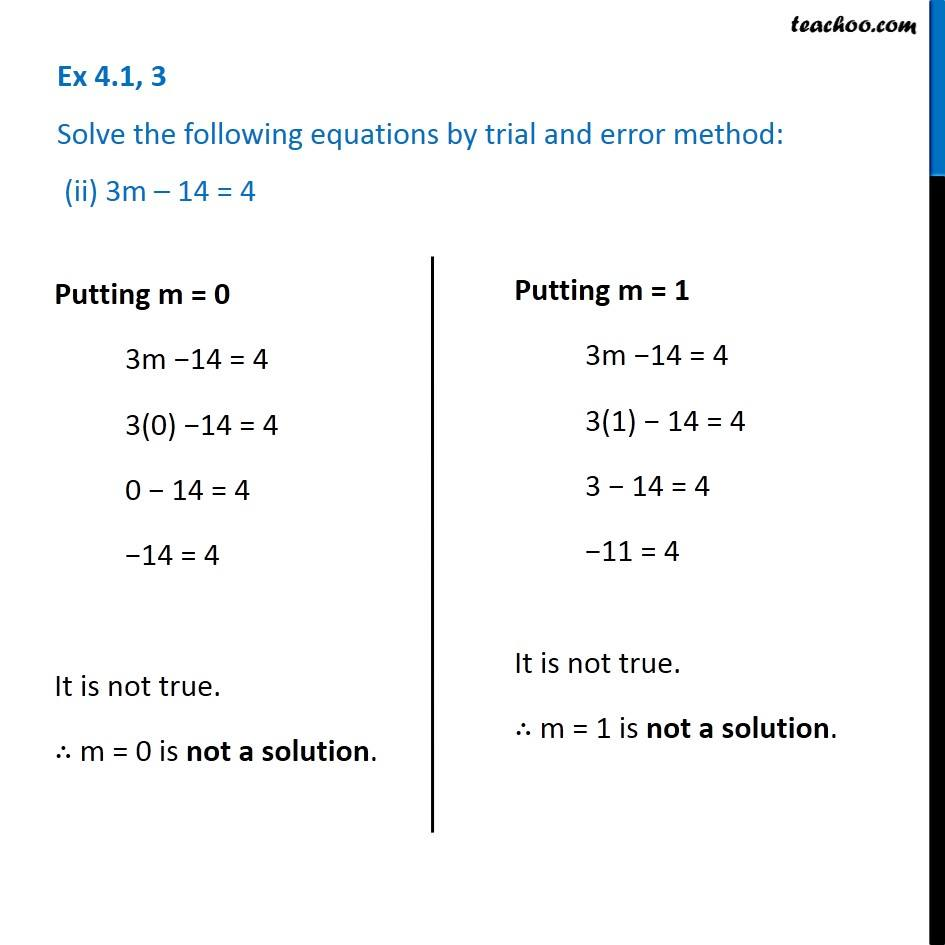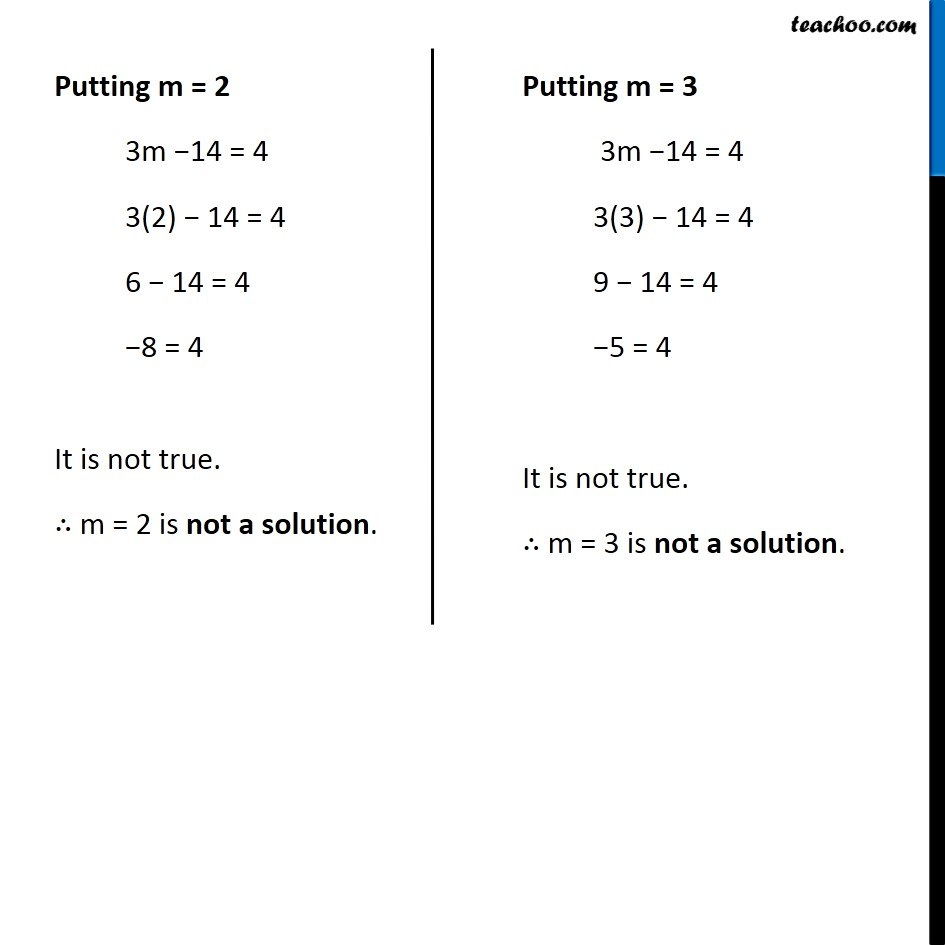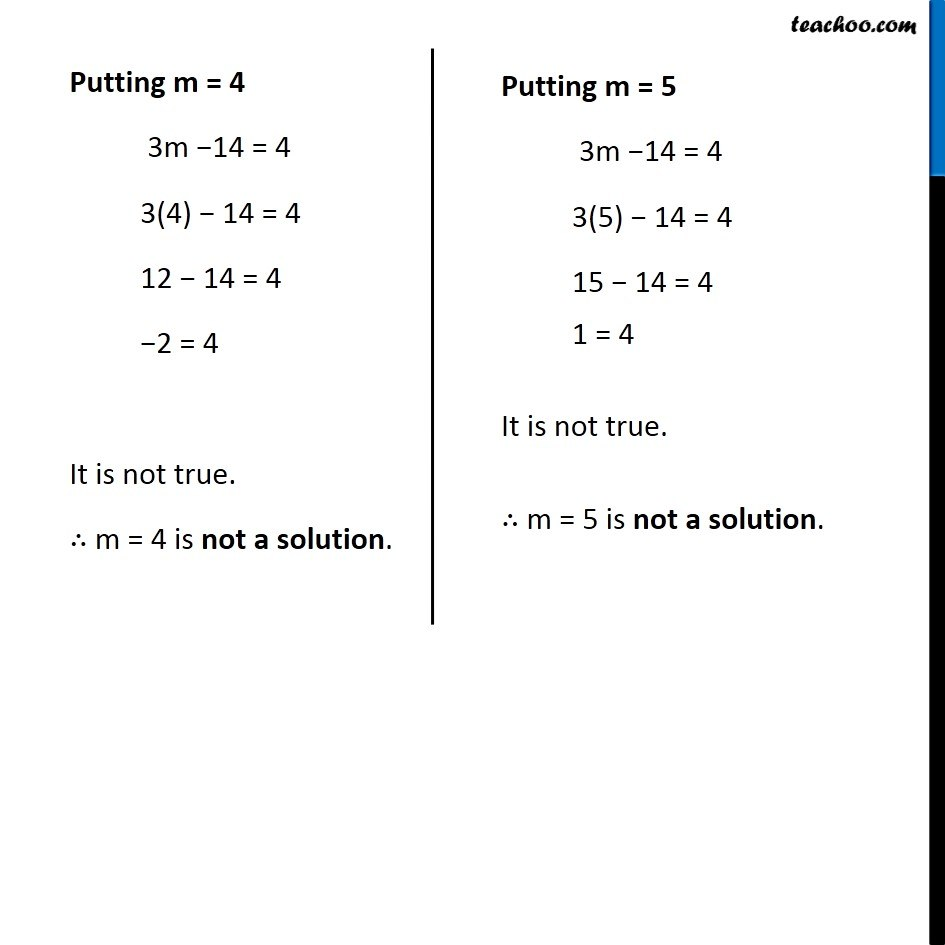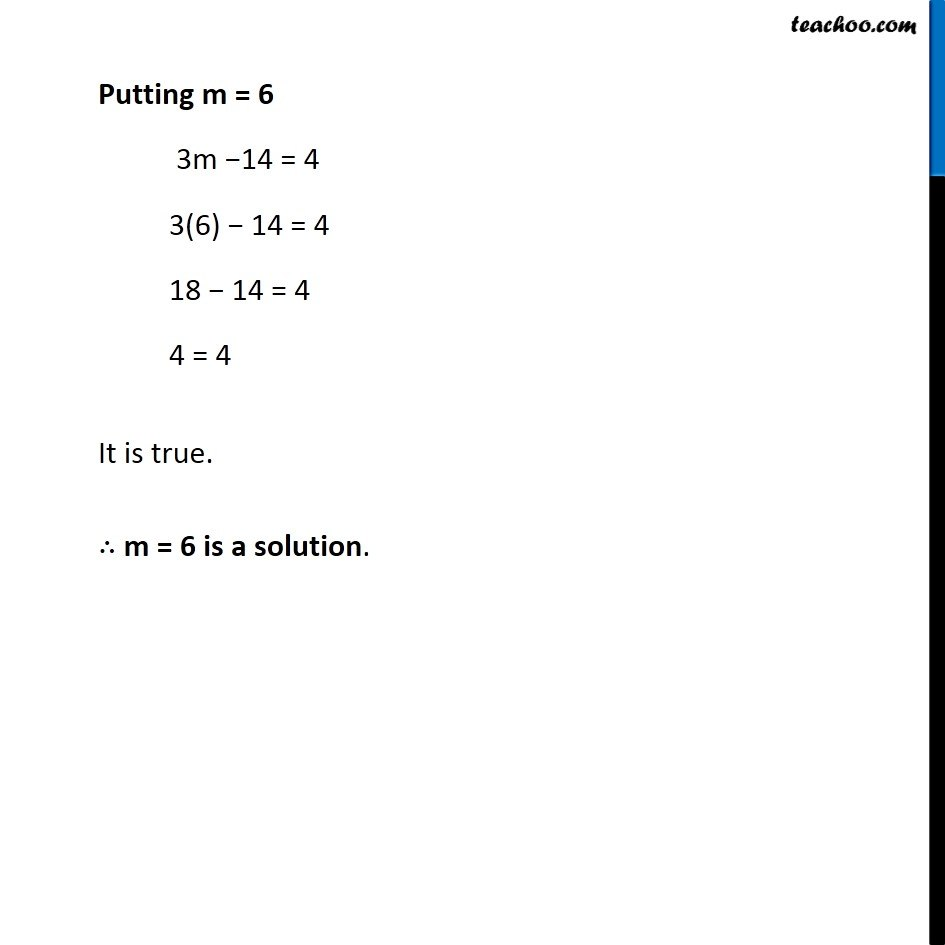1. Chapter 4 Class 7 Simple Equations
2. Concept wise
3. Solving Equations

Transcript

Ex 4.1, 3 Solve the following equations by trial and error method: (i) 5p + 2 = 17 Putting p = 0 5p + 2 = 17 5(0) + 2 = 17 0 + 2 = 17 2 = 17 It is not true. ∴ p = 0 is not a solution. Putting p = 1 5p + 2 = 17 5(1) + 2 = 17 5 + 2 = 17 7 = 17 It is not true. ∴ p = 1 is not a solution. Putting p = 2 5p + 2 = 17 5(2) + 2 = 17 10 + 2 = 17 12 = 17 It is not true. ∴ p = 1 is not a solution. Putting p = 3 5p + 2 = 17 5(3) + 2 = 17 15 + 2 = 17 17 = 17 It is true. ∴ p = 3 is a solution Ex 4.1, 3 Solve the following equations by trial and error method: (ii) 3m – 14 = 4 Putting m = 0 3m −14 = 4 3(0) −14 = 4 0 − 14 = 4 −14 = 4 It is not true. ∴ m = 0 is not a solution. Putting m = 1 3m −14 = 4 3(1) − 14 = 4 3 − 14 = 4 −11 = 4 It is not true. ∴ m = 1 is not a solution. Putting m = 2 3m −14 = 4 3(2) − 14 = 4 6 − 14 = 4 −8 = 4 It is not true. ∴ m = 2 is not a solution. Putting m = 3 3m −14 = 4 3(3) − 14 = 4 9 − 14 = 4 −5 = 4 It is not true. ∴ m = 3 is not a solution. Putting m = 4 3m −14 = 4 3(4) − 14 = 4 12 − 14 = 4 −2 = 4 It is not true. ∴ m = 4 is not a solution. Putting m = 5 3m −14 = 4 3(5) − 14 = 4 15 − 14 = 4 1 = 4 It is not true. ∴ m = 5 is not a solution. Putting m = 6 3m −14 = 4 3(6) − 14 = 4 18 − 14 = 4 4 = 4 It is true. ∴ m = 6 is a solution.

Solving Equations